#### Vol. 33, No. 1, 1970

 Download this articleFor screen For printingRecent Issues Vol. 325: 1 Vol. 324: 1  2 Vol. 323: 1  2 Vol. 322: 1  2 Vol. 321: 1  2 Vol. 320: 1  2 Vol. 319: 1  2 Vol. 318: 1  2Online Archive Volume: Issue:The Journal Subscriptions Editorial Board Officers Contacts Submission Guidelines Submission Form Policies for Authors ISSN: 1945-5844 (e-only) ISSN: 0030-8730 (print) Special Issues Author Index To Appear Other MSP Journals
On some extremal simplexes

### Mir Maswood Ali

Vol. 33 (1970), No. 1, 1–14
##### Abstract

Let A be a fixed point in n-dimensional Euclidean space. Let B1,B2,,Bn+1 be the vertices of a simplex Sn of n-dimensions, that is, the n + 1 vertices do not lie on a (n 1) dimensional subspace. Let di, assumed to be positive, be the distance of Bi from A, and let lij be the cosine of the angle between the straight lines ABi and ABj for i,j = 1,2, , n + 1. Let πi denote the (n 1)-dimensional hyperplane passing through all the vertices of Sn except Bi, let pi, assumed positive, be the perpendicular distance of πi from A, and let mij denote the cosine of the angle between the normals from A to πi and πj for i,j = 1,2,,n + 1. The present paper deals with the following problems.

(a) An expression for the content of Sn,C(Sn) say, in terms of di and lij for i,j = 1,2,,n + 1 is first obtained. Then leaving d1,d2,,dn+1 fixed, values of lij, say lij, are determined in such a manner that C(Sn) is a maximum, and the maximum value of C(Sn) is obtained for the two cases that arise: (i) when A is inside Sn, (ii) when A is outside Sn. The latter case does not arise when d1 = d2 = = dn+1.

(b) An expression for C(Sn) is obtained in terms of pi and mij,i,j = 1,2,,n + 1. Then leaving p1,p2,,pn+1 fixed, values for mij, say mij, are determined in such a manner that C(Sn) is a minimum, and such C(Sn) is computed for the two cases that arise depending on (i) whether A is inside Sn or (ii) A is outside Sn. The latter case does not arise when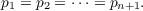The results are stated below.

(a) The content of Sn,maxC(Sn) and lij are given by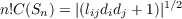(1.1)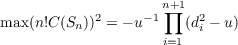(1.2)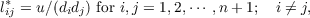(1.3)

where u satisfies the equation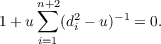(1.4)

The unique negative root for u in (1.4) corresponds to the case when A is inside Sn. When the relation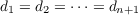is not satisfied, the smallest positive root for u in (1.4) corresponds to the case when A is outside Sn. Other roots for u in (1.4), if any, are inadmissible.

(b) The content iC(Sn), min(C(Sn)) and mij are given by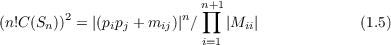where |Mii| is the cof actor of mii in |(mij)| and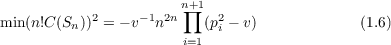and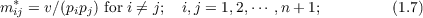where v satisfies the equation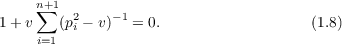The unique negative root for v in (1.8) corresponds to the case when A is inside Sn. When the relation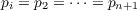is not satisfied, the smallest positive root for v in (1.8) corresponds to the case when A is outside Sn. All other roots, if any, are inadmissible.

When d1 = d2 = = dn+1, we obtain the special result that the largest simplex inscribed in a sphere of n-dimensions is a regular one, while when p1 = p2 = = pn+1 the smallest simplex circumscribing a sphere is a regular one.

Primary: 52.40
##### Milestones